# Mean State

Period Mean (original grids) [Watt m-2]
Model Period Mean (intersection) [Watt m-2]
Model Period Mean (complement) [Watt m-2]
Benchmark Period Mean (intersection) [Watt m-2]
Benchmark Period Mean (complement) [Watt m-2]
Bias [Watt m-2]
RMSE [Watt m-2]
Phase Shift [months]
Bias Score 
RMSE Score 
Seasonal Cycle Score 
Spatial Distribution Score 
Interannual Variability Score 
Overall Score 
Benchmark [-] 217.
CRUNCEPv7 [-] 228. 227. 0.00 217. 232. 11.0 22.1 0.909 0.58 0.47 0.87 0.99 0.52 0.65
GSWP3v1 [-] 217. 216. 0.00 217. 232. 0.504 14.1 0.318 0.86 0.54 0.96 1.0 0.76 0.78
WFDEI [-] 199. 198. 0.00 217. 232. -17.3 30.3 1.03 0.44 0.42 0.84 0.86 0.72 0.62
Period Mean (original grids) [Watt m-2]
Model Period Mean (intersection) [Watt m-2]
Model Period Mean (complement) [Watt m-2]
Benchmark Period Mean (intersection) [Watt m-2]
Benchmark Period Mean (complement) [Watt m-2]
Bias [Watt m-2]
RMSE [Watt m-2]
Phase Shift [months]
Bias Score 
RMSE Score 
Seasonal Cycle Score 
Spatial Distribution Score 
Interannual Variability Score 
Overall Score 
Benchmark [-] 241.
CRUNCEPv7 [-] 245. 245. 0.00 241. 238. 4.28 22.8 0.581 0.65 0.52 0.95 0.93 0.66 0.71
GSWP3v1 [-] 240. 241. 0.00 241. 238. -0.171 13.3 0.143 0.90 0.61 0.99 1.0 0.82 0.82
WFDEI [-] 236. 237. 0.00 241. 238. -4.14 24.3 0.456 0.65 0.50 0.96 0.75 0.77 0.69
Period Mean (original grids) [Watt m-2]
Model Period Mean (intersection) [Watt m-2]
Model Period Mean (complement) [Watt m-2]
Benchmark Period Mean (intersection) [Watt m-2]
Benchmark Period Mean (complement) [Watt m-2]
Bias [Watt m-2]
RMSE [Watt m-2]
Phase Shift [months]
Bias Score 
RMSE Score 
Seasonal Cycle Score 
Spatial Distribution Score 
Interannual Variability Score 
Overall Score 
Benchmark [-] 233.
CRUNCEPv7 [-] 231. 231. 0.00 233. 238. -1.27 21.2 0.855 0.73 0.55 0.89 0.91 0.55 0.70
GSWP3v1 [-] 233. 233. 0.00 233. 238. 0.143 15.9 0.237 0.89 0.60 0.97 0.99 0.70 0.79
WFDEI [-] 233. 233. 0.00 233. 238. -0.0886 23.3 0.790 0.76 0.51 0.90 0.95 0.67 0.72
Period Mean (original grids) [Watt m-2]
Model Period Mean (intersection) [Watt m-2]
Model Period Mean (complement) [Watt m-2]
Benchmark Period Mean (intersection) [Watt m-2]
Benchmark Period Mean (complement) [Watt m-2]
Bias [Watt m-2]
RMSE [Watt m-2]
Phase Shift [months]
Bias Score 
RMSE Score 
Seasonal Cycle Score 
Spatial Distribution Score 
Interannual Variability Score 
Overall Score 
Benchmark [-] 111.
CRUNCEPv7 [-] 112. 112. 0.00 111. 117. 0.518 16.3 0.0404 0.94 0.83 1.0 0.99 0.51 0.85
GSWP3v1 [-] 107. 107. 0.00 111. 117. -3.90 12.6 0.0241 0.95 0.85 1.0 0.90 0.69 0.87
WFDEI [-] 102. 102. 0.00 111. 117. -9.10 21.4 0.608 0.90 0.78 0.96 0.92 0.74 0.85
Period Mean (original grids) [Watt m-2]
Model Period Mean (intersection) [Watt m-2]
Model Period Mean (complement) [Watt m-2]
Benchmark Period Mean (intersection) [Watt m-2]
Benchmark Period Mean (complement) [Watt m-2]
Bias [Watt m-2]
RMSE [Watt m-2]
Phase Shift [months]
Bias Score 
RMSE Score 
Seasonal Cycle Score 
Spatial Distribution Score 
Interannual Variability Score 
Overall Score 
Benchmark [-] 213.
CRUNCEPv7 [-] 216. 215. 0.00 209. 225. 9.33 24.3 1.51 0.56 0.43 0.78 0.91 0.49 0.60
GSWP3v1 [-] 205. 205. 0.00 209. 225. 0.417 17.6 0.524 0.79 0.46 0.92 0.99 0.77 0.73
WFDEI [-] 190. 190. 0.00 209. 225. -14.3 27.6 1.23 0.50 0.41 0.81 0.96 0.63 0.62
Period Mean (original grids) [Watt m-2]
Model Period Mean (intersection) [Watt m-2]
Model Period Mean (complement) [Watt m-2]
Benchmark Period Mean (intersection) [Watt m-2]
Benchmark Period Mean (complement) [Watt m-2]
Bias [Watt m-2]
RMSE [Watt m-2]
Phase Shift [months]
Bias Score 
RMSE Score 
Seasonal Cycle Score 
Spatial Distribution Score 
Interannual Variability Score 
Overall Score 
Benchmark [-] 126.
CRUNCEPv7 [-] 134. 134. 0.00 126. 145. 7.98 18.7 0.157 0.89 0.82 0.99 1.0 0.58 0.85
GSWP3v1 [-] 126. 126. 0.00 126. 145. 0.0721 11.8 0.0425 0.98 0.86 1.0 1.0 0.64 0.89
WFDEI [-] 119. 118. 0.00 126. 145. -6.86 18.3 0.258 0.91 0.81 0.98 0.95 0.74 0.87
Period Mean (original grids) [Watt m-2]
Model Period Mean (intersection) [Watt m-2]
Model Period Mean (complement) [Watt m-2]
Benchmark Period Mean (intersection) [Watt m-2]
Benchmark Period Mean (complement) [Watt m-2]
Bias [Watt m-2]
RMSE [Watt m-2]
Phase Shift [months]
Bias Score 
RMSE Score 
Seasonal Cycle Score 
Spatial Distribution Score 
Interannual Variability Score 
Overall Score 
Benchmark [-] 170.
CRUNCEPv7 [-] 188. 187. 0.00 170. 169. 16.8 27.8 0.502 0.72 0.74 0.96 0.89 0.62 0.78
GSWP3v1 [-] 171. 171. 0.00 170. 169. 0.441 14.1 0.129 0.95 0.79 0.99 1.0 0.62 0.85
WFDEI [-] 180. 179. 0.00 170. 169. 8.92 24.9 0.541 0.82 0.70 0.95 0.95 0.69 0.80
Period Mean (original grids) [Watt m-2]
Model Period Mean (intersection) [Watt m-2]
Model Period Mean (complement) [Watt m-2]
Benchmark Period Mean (intersection) [Watt m-2]
Benchmark Period Mean (complement) [Watt m-2]
Bias [Watt m-2]
RMSE [Watt m-2]
Phase Shift [months]
Bias Score 
RMSE Score 
Seasonal Cycle Score 
Spatial Distribution Score 
Interannual Variability Score 
Overall Score 
Benchmark [-] 210.
CRUNCEPv7 [-] 231. 231. 0.00 209. 224. 21.5 32.3 0.302 0.56 0.59 0.98 0.99 0.54 0.71
GSWP3v1 [-] 208. 208. 0.00 209. 224. 0.482 16.5 0.148 0.89 0.66 0.99 0.99 0.66 0.81
WFDEI [-] 207. 207. 0.00 209. 224. -1.19 28.0 0.414 0.70 0.55 0.97 0.82 0.65 0.71
Period Mean (original grids) [Watt m-2]
Model Period Mean (intersection) [Watt m-2]
Model Period Mean (complement) [Watt m-2]
Benchmark Period Mean (intersection) [Watt m-2]
Benchmark Period Mean (complement) [Watt m-2]
Bias [Watt m-2]
RMSE [Watt m-2]
Phase Shift [months]
Bias Score 
RMSE Score 
Seasonal Cycle Score 
Spatial Distribution Score 
Interannual Variability Score 
Overall Score 
Benchmark [-] 105.
CRUNCEPv7 [-] 103. 104. 0.00 105. 108. -1.87 18.0 0.284 0.92 0.80 0.98 0.90 0.52 0.82
GSWP3v1 [-] 96.3 96.5 0.00 105. 108. -8.76 12.5 0.0632 0.90 0.83 1.0 0.80 0.64 0.83
WFDEI [-] 89.7 89.9 0.00 105. 108. -15.3 20.2 0.176 0.84 0.78 0.99 0.97 0.70 0.84
Period Mean (original grids) [Watt m-2]
Model Period Mean (intersection) [Watt m-2]
Model Period Mean (complement) [Watt m-2]
Benchmark Period Mean (intersection) [Watt m-2]
Benchmark Period Mean (complement) [Watt m-2]
Bias [Watt m-2]
RMSE [Watt m-2]
Phase Shift [months]
Bias Score 
RMSE Score 
Seasonal Cycle Score 
Spatial Distribution Score 
Interannual Variability Score 
Overall Score 
Benchmark [-] 228.
CRUNCEPv7 [-] 235. 235. 0.00 229. 216. 2.90 18.7 0.429 0.88 0.74 0.97 0.97 0.65 0.82
GSWP3v1 [-] 232. 232. 0.00 229. 216. 0.0913 15.0 0.0879 0.95 0.76 0.99 1.0 0.68 0.86
WFDEI [-] 237. 237. 0.00 229. 216. 4.10 24.4 0.294 0.88 0.65 0.98 1.0 0.80 0.83
Period Mean (original grids) [Watt m-2]
Model Period Mean (intersection) [Watt m-2]
Model Period Mean (complement) [Watt m-2]
Benchmark Period Mean (intersection) [Watt m-2]
Benchmark Period Mean (complement) [Watt m-2]
Bias [Watt m-2]
RMSE [Watt m-2]
Phase Shift [months]
Bias Score 
RMSE Score 
Seasonal Cycle Score 
Spatial Distribution Score 
Interannual Variability Score 
Overall Score 
Benchmark [-] 140.
CRUNCEPv7 [-] 158. 158. 0.00 138. 161. 20.2 28.5 0.194 0.75 0.80 0.99 1.0 0.64 0.83
GSWP3v1 [-] 135. 135. 0.00 138. 161. 0.0454 13.3 0.0808 0.95 0.85 0.99 1.0 0.64 0.88
WFDEI [-] 136. 136. 0.00 138. 161. 0.763 21.0 0.264 0.91 0.78 0.98 0.98 0.77 0.86
Period Mean (original grids) [Watt m-2]
Model Period Mean (intersection) [Watt m-2]
Model Period Mean (complement) [Watt m-2]
Benchmark Period Mean (intersection) [Watt m-2]
Benchmark Period Mean (complement) [Watt m-2]
Bias [Watt m-2]
RMSE [Watt m-2]
Phase Shift [months]
Bias Score 
RMSE Score 
Seasonal Cycle Score 
Spatial Distribution Score 
Interannual Variability Score 
Overall Score 
Benchmark [-] 191.
CRUNCEPv7 [-] 191. 191. 0.00 186. 193. 4.01 23.2 0.455 0.76 0.69 0.95 0.98 0.54 0.77
GSWP3v1 [-] 182. 182. 0.00 186. 193. -4.43 14.4 0.132 0.91 0.73 0.99 0.99 0.66 0.83
WFDEI [-] 184. 184. 0.00 186. 193. -3.04 24.7 0.454 0.77 0.65 0.95 0.98 0.70 0.78
Period Mean (original grids) [Watt m-2]
Model Period Mean (intersection) [Watt m-2]
Model Period Mean (complement) [Watt m-2]
Benchmark Period Mean (intersection) [Watt m-2]
Benchmark Period Mean (complement) [Watt m-2]
Bias [Watt m-2]
RMSE [Watt m-2]
Phase Shift [months]
Bias Score 
RMSE Score 
Seasonal Cycle Score 
Spatial Distribution Score 
Interannual Variability Score 
Overall Score 
Benchmark [-] 240.
CRUNCEPv7 [-] 249. 249. 0.00 240. 241. 8.59 22.4 0.367 0.81 0.74 0.97 0.99 0.61 0.81
GSWP3v1 [-] 240. 240. 0.00 240. 241. -0.0717 13.2 0.119 0.94 0.79 0.99 1.0 0.62 0.86
WFDEI [-] 255. 254. 0.00 240. 241. 13.5 23.2 0.392 0.78 0.73 0.97 1.0 0.58 0.80
Period Mean (original grids) [Watt m-2]
Model Period Mean (intersection) [Watt m-2]
Model Period Mean (complement) [Watt m-2]
Benchmark Period Mean (intersection) [Watt m-2]
Benchmark Period Mean (complement) [Watt m-2]
Bias [Watt m-2]
RMSE [Watt m-2]
Phase Shift [months]
Bias Score 
RMSE Score 
Seasonal Cycle Score 
Spatial Distribution Score 
Interannual Variability Score 
Overall Score 
Benchmark [-] 113.
CRUNCEPv7 [-] 117. 117. 0.00 113. 116. 3.88 18.4 0.110 0.89 0.82 0.99 0.79 0.48 0.80
GSWP3v1 [-] 108. 108. 0.00 113. 116. -5.10 12.3 0.0429 0.94 0.85 1.0 0.85 0.72 0.86
WFDEI [-] 107. 107. 0.00 113. 116. -5.88 21.3 0.320 0.91 0.78 0.98 0.85 0.73 0.84
Period Mean (original grids) [Watt m-2]
Model Period Mean (intersection) [Watt m-2]
Model Period Mean (complement) [Watt m-2]
Benchmark Period Mean (intersection) [Watt m-2]
Benchmark Period Mean (complement) [Watt m-2]
Bias [Watt m-2]
RMSE [Watt m-2]
Phase Shift [months]
Bias Score 
RMSE Score 
Seasonal Cycle Score 
Spatial Distribution Score 
Interannual Variability Score 
Overall Score 
Benchmark [-] 229.
CRUNCEPv7 [-] 223. 223. 0.00 227. 241. -2.57 26.4 0.757 0.67 0.63 0.92 0.70 0.57 0.69
GSWP3v1 [-] 226. 226. 0.00 227. 241. 0.355 16.5 0.210 0.85 0.68 0.98 0.99 0.69 0.81
WFDEI [-] 228. 228. 0.00 227. 241. -0.377 24.8 0.346 0.72 0.62 0.96 0.97 0.67 0.76
Period Mean (original grids) [Watt m-2]
Model Period Mean (intersection) [Watt m-2]
Model Period Mean (complement) [Watt m-2]
Benchmark Period Mean (intersection) [Watt m-2]
Benchmark Period Mean (complement) [Watt m-2]
Bias [Watt m-2]
RMSE [Watt m-2]
Phase Shift [months]
Bias Score 
RMSE Score 
Seasonal Cycle Score 
Spatial Distribution Score 
Interannual Variability Score 
Overall Score 
Benchmark [-] 173.
CRUNCEPv7 [-] 186. 186. 0.00 172. 207. 15.1 22.2 0.367 0.77 0.78 0.98 0.98 0.50 0.80
GSWP3v1 [-] 171. 171. 0.00 172. 207. 0.686 13.8 0.0926 0.96 0.80 0.99 1.0 0.63 0.87
WFDEI [-] 181. 181. 0.00 172. 207. 10.3 22.6 0.247 0.84 0.73 0.98 1.0 0.75 0.84
Period Mean (original grids) [Watt m-2]
Model Period Mean (intersection) [Watt m-2]
Model Period Mean (complement) [Watt m-2]
Benchmark Period Mean (intersection) [Watt m-2]
Benchmark Period Mean (complement) [Watt m-2]
Bias [Watt m-2]
RMSE [Watt m-2]
Phase Shift [months]
Bias Score 
RMSE Score 
Seasonal Cycle Score 
Spatial Distribution Score 
Interannual Variability Score 
Overall Score 
Benchmark [-] 135.
CRUNCEPv7 [-] 149. 149. 0.00 135. 133. 13.8 20.4 0.186 0.83 0.82 0.99 0.96 0.54 0.83
GSWP3v1 [-] 135. 135. 0.00 135. 133. 0.0758 11.1 0.0540 0.98 0.86 1.0 1.0 0.65 0.89
WFDEI [-] 137. 137. 0.00 135. 133. 1.93 18.1 0.157 0.93 0.80 0.99 0.86 0.82 0.87
Period Mean (original grids) [Watt m-2]
Model Period Mean (intersection) [Watt m-2]
Model Period Mean (complement) [Watt m-2]
Benchmark Period Mean (intersection) [Watt m-2]
Benchmark Period Mean (complement) [Watt m-2]
Bias [Watt m-2]
RMSE [Watt m-2]
Phase Shift [months]
Bias Score 
RMSE Score 
Seasonal Cycle Score 
Spatial Distribution Score 
Interannual Variability Score 
Overall Score 
Benchmark [-] 193.
CRUNCEPv7 [-] 191. 191. 0.00 193. 181. -1.86 16.9 0.233 0.92 0.81 0.98 0.98 0.54 0.84
GSWP3v1 [-] 193. 193. 0.00 193. 181. -0.0247 13.4 0.0748 0.97 0.84 1.0 1.0 0.65 0.88
WFDEI [-] 208. 208. 0.00 193. 181. 14.7 25.4 0.164 0.82 0.77 0.99 0.99 0.78 0.85
Period Mean (original grids) [Watt m-2]
Model Period Mean (intersection) [Watt m-2]
Model Period Mean (complement) [Watt m-2]
Benchmark Period Mean (intersection) [Watt m-2]
Benchmark Period Mean (complement) [Watt m-2]
Bias [Watt m-2]
RMSE [Watt m-2]
Phase Shift [months]
Bias Score 
RMSE Score 
Seasonal Cycle Score 
Spatial Distribution Score 
Interannual Variability Score 
Overall Score 
Benchmark [-] 202.
CRUNCEPv7 [-] 211. 211. 0.00 201. 241. 10.1 21.6 1.24 0.61 0.46 0.83 0.91 0.51 0.63
GSWP3v1 [-] 201. 201. 0.00 201. 241. 0.303 15.0 0.334 0.86 0.50 0.96 0.99 0.75 0.76
WFDEI [-] 184. 184. 0.00 201. 241. -17.2 28.1 1.36 0.44 0.42 0.82 0.97 0.59 0.61
Period Mean (original grids) [Watt m-2]
Model Period Mean (intersection) [Watt m-2]
Model Period Mean (complement) [Watt m-2]
Benchmark Period Mean (intersection) [Watt m-2]
Benchmark Period Mean (complement) [Watt m-2]
Bias [Watt m-2]
RMSE [Watt m-2]
Phase Shift [months]
Bias Score 
RMSE Score 
Seasonal Cycle Score 
Spatial Distribution Score 
Interannual Variability Score 
Overall Score 
Benchmark [-] 211.
CRUNCEPv7 [-] 217. 217. 0.00 211. 216. 5.51 23.6 0.836 0.77 0.58 0.90 0.84 0.49 0.69
GSWP3v1 [-] 212. 212. 0.00 211. 216. 0.782 17.8 0.173 0.92 0.62 0.98 1.0 0.67 0.80
WFDEI [-] 204. 204. 0.00 211. 216. -6.94 25.6 0.495 0.77 0.54 0.95 1.0 0.75 0.76
Period Mean (original grids) [Watt m-2]
Model Period Mean (intersection) [Watt m-2]
Model Period Mean (complement) [Watt m-2]
Benchmark Period Mean (intersection) [Watt m-2]
Benchmark Period Mean (complement) [Watt m-2]
Bias [Watt m-2]
RMSE [Watt m-2]
Phase Shift [months]
Bias Score 
RMSE Score 
Seasonal Cycle Score 
Spatial Distribution Score 
Interannual Variability Score 
Overall Score 
Benchmark [-] 204.
CRUNCEPv7 [-] 210. 209. 0.00 205. 190. 1.38 32.0 0.437 0.70 0.69 0.96 0.76 0.52 0.72
GSWP3v1 [-] 210. 208. 0.00 205. 190. -0.797 19.6 0.252 0.88 0.73 0.97 1.0 0.64 0.82
WFDEI [-] 215. 215. 0.00 205. 190. 5.63 31.7 0.662 0.75 0.66 0.93 0.98 0.66 0.77

# Temporally integrated period mean

BENCHMARK MEAN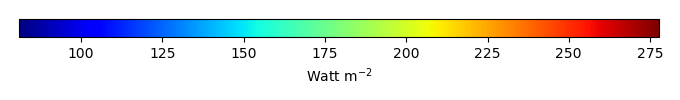MODEL MEANBIAS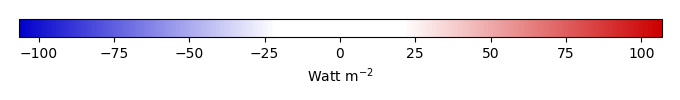BIAS SCORERMSE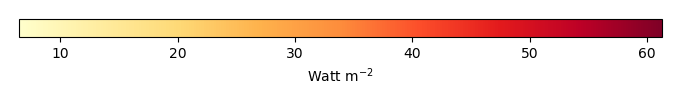RMSE SCOREBENCHMARK INTERANNUAL VARIABILITY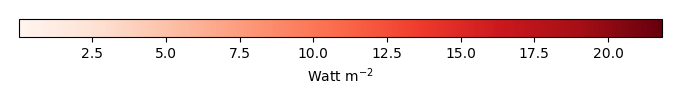MODEL INTERANNUAL VARIABILITYINTERANNUAL VARIABILITY SCOREBENCHMARK MAX MONTHMODEL MAX MONTHDIFFERENCE IN MAX MONTH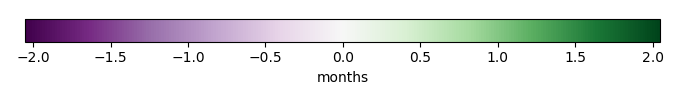SEASONAL CYCLE SCORESPATIAL TAYLOR DIAGRAMMODEL COLORS# Spatially integrated regional mean

MODEL COLORSREGIONAL MEANANNUAL CYCLEMONTHLY ANOMALYANNUAL CYCLE# All Models

BenchmarkCRUNCEPv7GSWP3v1WFDEI# Data Information

creation_date: Mon Jun 30 23:16:59 PDT 2014

source_file: This product is generated from monthly 1 degree GEWEX SRB Radiation observations